# Calculate Quarter from a Date – Excel & Google Sheets

This tutorial will demonstrate how to calculate the quarter from a date, including how to calculate the current quarter.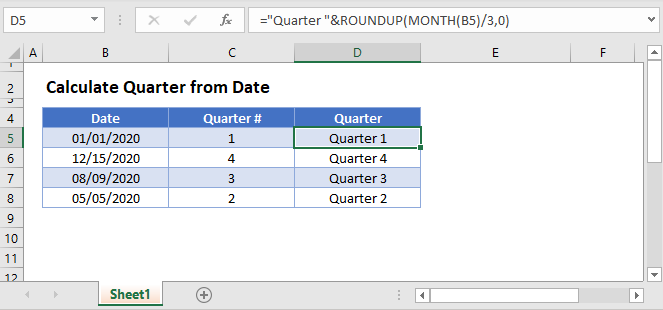## Quarter of a Date

To calculate the quarter from a date, first we want to get the month number:

``=MONTH(B3)``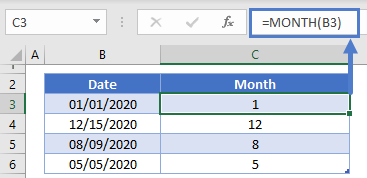Next we want to divide the month number by 3 (each quarter has 3 months) and use the ROUNDUP Function to round the result up to the nearest whole integer:

``=ROUNDUP(MONTH(B3)/3,0)``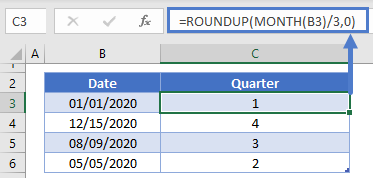Next, we can concatenate text to the front of the result to say Q1, Q2, Q3, or Q4:

``=CONCATENATE("Q",ROUNDUP(MONTH(B3)/3,0))``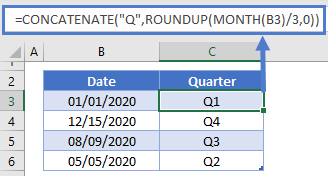or “Quarter 1”, “Quarter 2”, etc.

``=CONCATENATE("Quarter",ROUNDUP(MONTH(B3)/3,0))``

## Calculate Current Quarter

To calculate the current quarter, simply use the TODAY or NOW functions to get the current date for use in one of the above formulas.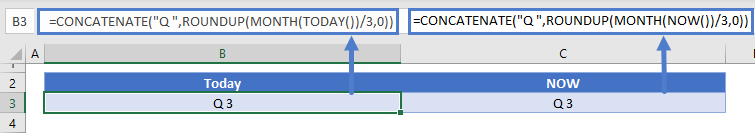## Calculate Quarter from a Date in Google Sheets

All of the above examples work exactly the same in Google Sheets as in Excel.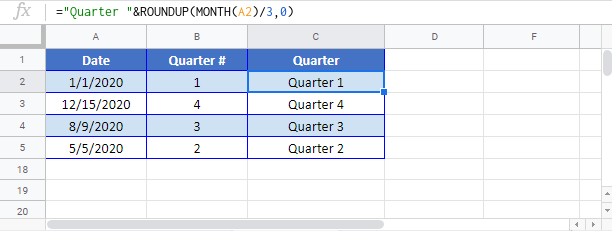### Excel Practice Worksheet

Practice Excel functions and formulas with our 100% free practice worksheets!